# 基于周期性多态洗衣机运行的混合事件检测算法Hybrid Event Detection Algorithm Based on Periodic Polymorphic Washing Machine Operation

DOI: 10.12677/SG.2021.113023, PDF, HTML, XML, 下载: 18  浏览: 56

Abstract: Event detection is an important part of non-intrusive load monitoring and identification. Aiming at the problem that multiple events cannot be recognized during the operation of washing machine, this paper proposes a mixed event detection algorithm based on the operation of periodic polymorphic washing machine. Firstly, the start and stop waveform is captured by the CUSUM algorithm, and the shapeDTW algorithm is introduced to match and identify the periodic waveform, and it is stripped from the total load data. The method can avoid the influence of periodic waveform on the detection of other electrical events and has high adaptive ability to identify unknown periodic waveform. In the case of multi-state washing machine participating in the operation, the simulation analysis and method comparison of various characteristic loads are carried out. The algorithm ensures the accuracy of feature extraction and has high detection accuracy, which provides a reference for the optimization of event detection algorithm.

1. 引言

2. 多负荷同时发生状态变化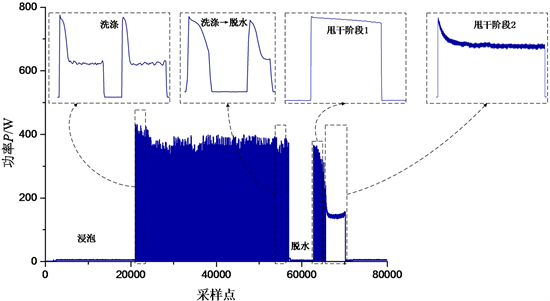Figure 1. The power curve of washing machine operation

3. 混合事件检测算法

3.1. CUSUM事件检测算法

${M}_{m}=\frac{\underset{j=k}{\overset{k+m-1}{\sum }}i\left(j\right)}{m}$ (1)

${M}_{d}=\frac{\underset{j=k+m}{\overset{k+m+n-1}{\sum }}i\left(j\right)}{n}$ (2)

${g}_{k}^{+}=\mathrm{max}\left\{0,{g}_{k-1}^{+}+{M}_{d}-\left({M}_{m}+\beta \right)\right\},{g}_{0}^{+}=0$ (3)

${g}_{k}^{-}=\mathrm{max}\left\{0,{g}_{k-1}^{-}-{M}_{d}+\left({M}_{m}-\beta \right)\right\},{g}_{0}^{-}=0$ (4)

3.2. shapeDTW算法

XY之间的时间弯曲路径为

$p=\left({p}_{1},{p}_{2},\cdots ,{p}_{k},\cdots ,{p}_{l}\right)$ (5)

$\left\{\begin{array}{l}{p}_{1}=\left(1,1\right),{p}_{l}=\left(i,j\right)\\ {i}_{1}\le {i}_{2}\le \cdot \cdot \cdot \le {i}_{l},{j}_{1}\le {j}_{2}\le \cdot \cdot \cdot \le {j}_{l}\\ {p}_{k+1}-{p}_{k}\in \left\{\left(1,1\right),\left(0,1\right),\left(1,0\right)\right\}\end{array}$ (6)

$D\left(i,j\right)=C\left(i,j\right)+\mathrm{min}\left\{D\left(i-1,j\right),D\left(i-1,j-1\right),D\left(i,j-1\right)\right\}$ (7)

DTW算法在某些约束条件下找到了全局最优的匹配，但它不一定能实现局部合理的匹配。因此在实际应用时会存在一定的误判情况，原因之一是因为DTW算法为寻求全局最优解而过度拉伸和缩放 ，如附图2(a)所示。为了解决这个问题，本文引入了shapeDTW算法 。shapeDTW算法先针对每个时序数据点，利用其前序和后序的部分数据提取出其局部形态特征，再将每个点周围的局部形状信息结合到动态规划匹配过程中，从而产生更正确的对齐结果，即具有相似局部形状的点倾向于被匹配，而具有不相似领域的点不太可能被匹配。相比于标准的DTW算法只关注全局最优解，shapeDTW算法更加关注时间序列在局部形态特征上的匹配长度。由此可见，shapeDTW算法可以利用局部形态特征，在很大程度上避免了过度拉伸和缩放所导致的误判问题。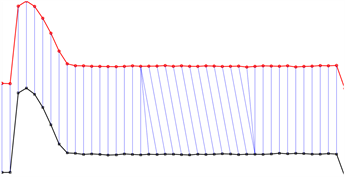Figure 2. DTW algorithm principle schematic diagram

3.3. 建立自适应周期性波形数据库

3.3.1. 波形聚类

$E=\underset{i=1}{\overset{K}{\sum }}\underset{j\in {C}_{i}}{\overset{}{\sum }}\text{DTW}\left({z}_{j}-{m}_{i}\right)$ (8)

3.3.2. 自适应周期性波形数据库

3.4. 混合事件检测算法流程

1) 输入总负荷电流数据。通常事件检测考虑电流与功率特征，由于电流数据更加稳定，本文取电流为被检测的电气量。

2) 检测启停波形。CUSUM_1用于检测周期性波形的启停，由于洗衣进周期性波形的启停间隔较短，因此需设置较小的窗口。

3) 识别周期性波形。将2)中检测到的启停波形进行shapeDTW匹配，若出现连续3次波形匹配为同一个电器，则认为此时打开了该电器。当判断有周期性波形设备打开时，则将同类波形删除，即将连续检测到的启–停位置之间的数据剥离。最后，将各个片段重新连接成一个新的时间序列。

4) 检测其他电器波形。CUSUM_2用于检测3)处理后的数据。此时可认为无周期性电器运行，即SCP成立。因此窗口设置较大。具体设置可参考案例中电器的事件间隔大小。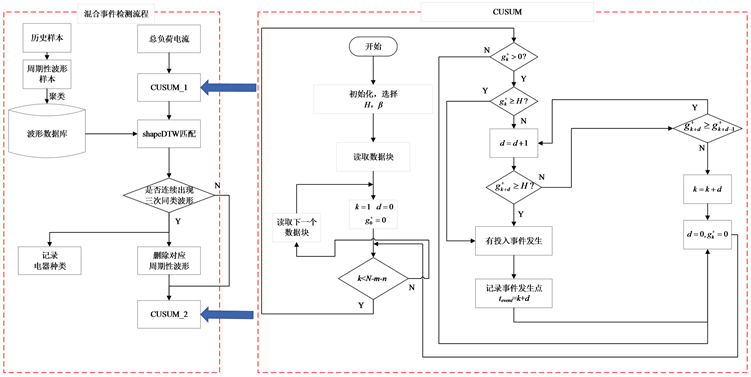Figure 3. Mixed event detection algorithm flow chart

4. 试验与分析

4.1. 参数设置

4.1.1. 窗口设置

CUSUM_1负责检测周期负荷事件，窗口长度设置较小，取均值计算窗口长度m为2，检测窗口长度n为2。本算例除洗衣机外，事件发生间隔大多数在50个采样点以上，因此CUSUM_2窗口长度设置为50，取均值计算窗口长度m为40，检测窗口长度n为10。shapeDTW算法中形状特征采用一维的梯度直方图 。对比算法CUSUM和GLR算法的窗口均设置为50。

4.1.1. 阈值设置

4.2. 评价指标

$\text{Precision}=\frac{|TP|}{|TP|+|FP|}$ (9)

$\text{Recall}=\frac{|TP|}{|TP|+|FN|}$ (10)

$\text{F_Score}=\frac{2}{\frac{1}{\text{Precision}}+\frac{1}{\text{Recall}}}$ (11)

4.3. 实例1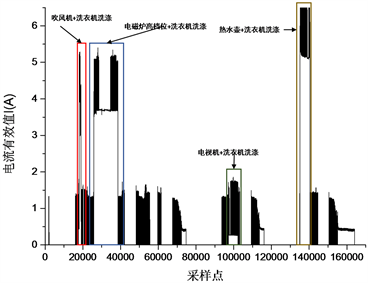(a)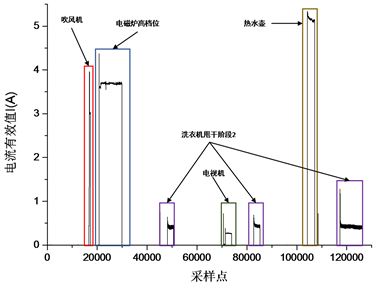(b)

Figure 4. RMS curve of mixed operation current of washing machine, hair dryer, induction cooker, TV set and hot kettleTable 1. Results of event detectionTable 2. Operating power of hair dryer, induction cooker, TV set and hot kettleTable 3. Comparison of feature extraction results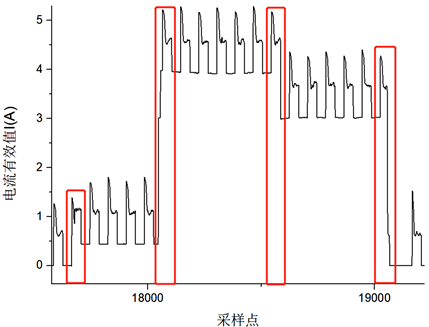Figure 5. The current effective value curve of hair dryer and washing machine in mixed operation

4.4. 实例2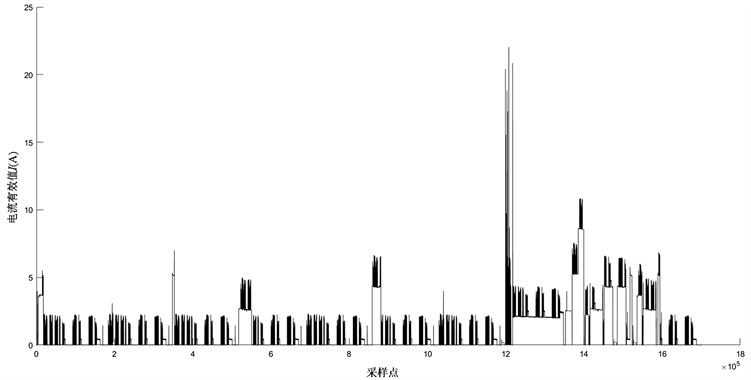Figure 6. Current effective value curveTable 4. Results of event detection

5. 结论

1) 在洗衣机运行背景下，就对特征提取的影响而言，相比于CUSUM和GLR算法，混合事件检测算法可保证提取的差量特征的准确性，为后续的特征提取、负荷辨识等工作打下了基础。

2) 在洗衣机运行背景下，就检测效果而言，混合事件检测算法较CUSUM和GLR算法具有更高的精确率。因此更适用于用户非侵入式负荷监测的工程实际。

1. 正文图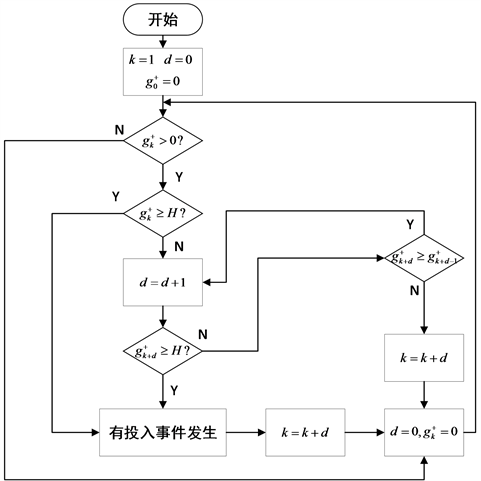Figure A1. Sliding window bilateral CUSUM event detection algorithm flow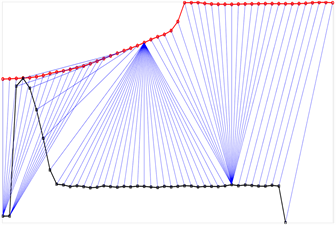(a) DTW(b) shapeDTW

Figure A2. Comparison of DTW and SHAPEDTW matching paths

2. 建立自适应波形数据库

1) 周期性波形数据库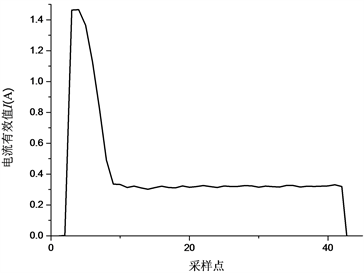(a) 洗衣机洗涤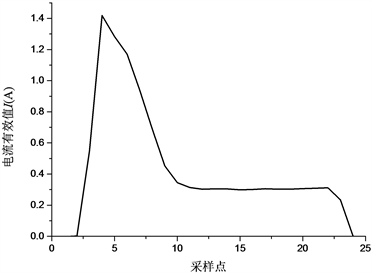(b) 洗衣机洗涤→脱水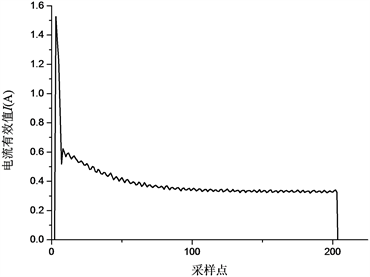(c) 洗衣机甩干1阶段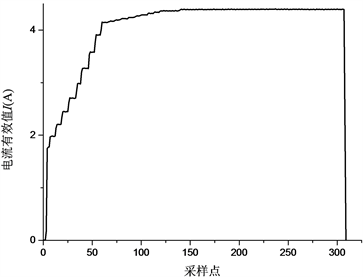(d) 电磁炉文火挡位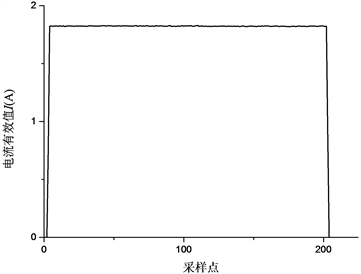(e) 电饭煲保温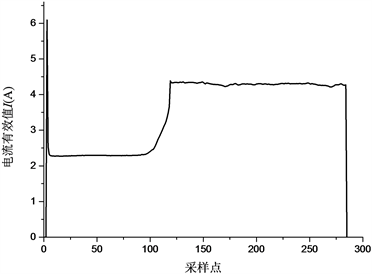(f) 微波炉低档位

Figure A3. Periodic waveform database

2) 检测周期性波形流程

① 通过CUSUM_1检测完整的启停波形，如附图4所示。

② 将检测到的波形进行shapeDTW匹配，以附图4波形为例，shapeDTW匹配结果如附表1所示。

③ 若出现连续3次波形匹配为同一个电器，则认为此时打开了该电器。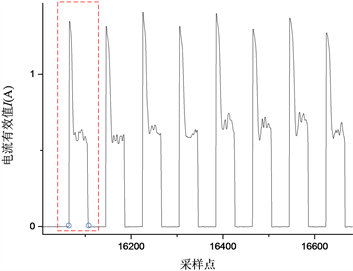Figure A4. Start and stop waveform detection in washing machineTable A1. Matching results of shapeDTW

3. 阈值训练

1) Hh训练过程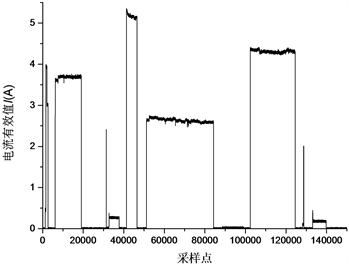Figure A5. Current effective value curve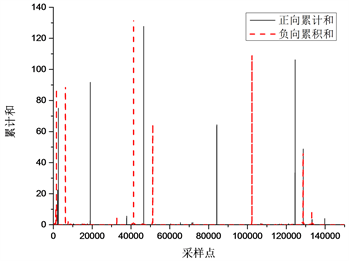Figure A6. Test process accumulation and schematic diagramTable A2. Event detection results of CUSUMTable A3. Event detection results of GLR

2) T的训练过程

T的取值应至少满足：同类波形到聚类中心的DTW距离Tr小于T。如式(12)所示。

$\begin{array}{l}T=\mathrm{max}\left({T}_{r}\right),r=1,2,3,4,5,6\\ {T}_{r}=\underset{j\in {C}_{i}}{\mathrm{max}}\left(\text{DTW}\left({z}_{j}-{m}_{i}\right)\right)\end{array}$ (12)Table A4. Tr distances for different waveforms

  Hart, G.W. (1992) Nonintrusive Appliance Load Monitoring. Proceedings of the IEEE, 80, 1870-1891. https://doi.org/10.1109/5.192069  Weiss, M., Helfenstein, A., Mattern, F. and Staake, T. (2012) Leveraging Smart Meter Data to Recognize Home Appliances. 2012 IEEE International Conference on Pervasive Computing & Communications, Lugano, 19-23 March 2012, 190-197. https://doi.org/10.1109/PerCom.2012.6199866  张露, Auger, F., 荆朝霞, Houidi, S., Bui, H.K., 肖江. 基于非侵入式的事件检测方法统计评估[J]. 电测与仪表, 2020, 57(1): 106-112, 120.  Berges, M., Goldman, E., Scott Matthews, H. and Soibelman, L. (2009) Learning Systems for Electric Consumption of Buildings. Proceedings of 2009 ASCE International Workshop on Computing in Civil Engineering, Austin, 24-27 June 2009, 1-10. https://doi.org/10.1061/41052(346)1  Yang, C.C., Soh, C.S. and Yap, V.V. (2014) Comparative Study of Event Detection Methods for Non-Intrusive Appliance Load Monitoring. Energy Procedia, 61, 1840-1843. https://doi.org/10.1016/j.egypro.2014.12.225  Tsai, M. and Lin, Y. (2012) Modern Development of an Adaptive Non-Intrusive Appliance Load Monitoring System in Electricity Energy Conservation. Applied Energy, 96, 55-73. https://doi.org/10.1016/j.apenergy.2011.11.027  De Baets, L., Ruyssinck, J., Develder, C., Dhaene, T. and Deschrijver, D. (2017) On the Bayesian Optimization and Robustness of Event Detection Methods in NILM. Energy and Buildings, 145, 57-66. https://doi.org/10.1016/j.enbuild.2017.03.061  Jin, Y., et al. (2011) A Time-Frequency Approach for Event Detection in Non-Intrusive Load Monitoring, SPIE Defense, Security, and Sensing. International Society for Optics and Photonics, 80501U-80501U. https://doi.org/10.1117/12.884385  Yang, C.C., Soh, C.S. and Yap, V.V. (2015) A Systematic Approach to ON-OFF Event Detection and Clustering Analysis of Non-Intrusive Appliance Load Monitoring. Frontiers in Energy, 9, 231-237. https://doi.org/10.1007/s11708-015-0358-6  肖江, Auger, F., 荆朝霞, Houidi, S. 基于贝叶斯信息准则的非侵入式负荷事件检测算法[J]. 电力系统保护与控制, 2018, 46(22): 8-14.  陈中, 方国权, 赵家庆, 丁宏恩. 基于贝叶斯迭代的非侵入式负荷事件检测方法[J]. 电测与仪表, 2021, 58(4): 1-8.  Zhu, Z., Zhang, S., Wei, Z., Yin, B. and Huang, X. (2018) A Novel CUSUM-Based Approach for Event Detection in Smart Metering. IOP Conference Series: Materials Science and Engineering, 322, Article ID: 072014. https://doi.org/10.1088/1757-899X/322/7/072014  牛卢璐, 贾宏杰. 一种适用于非侵入式负荷监测的暂态事件检测算法[J]. 电力系统自动化, 2011, 35(9): 30-35.  史帅彬, 张恒, 邓世聪, 周东国, 周洪, 胡文山. 基于复合滑动窗的CUSUM暂态事件检测算法[J]. 电测与仪表, 2019, 56(17): 13-18.  Lu, M. and Li, Z. (2019) A Hybrid Event Detection Approach for Non-Intrusive Load Monitoring. IEEE Transactions on Smart Grid, 11, 528-540. https://doi.org/10.1109/TSG.2019.2924862  徐志翔. 基于机器学习的非侵入式负荷监测技术研究[D]: [硕士学位论文]. 杭州: 浙江大学, 2020.  郭红霞, 陆进威, 杨苹, 刘泽健. 非侵入式负荷监测关键技术问题研究综述[J/OL]. 电力自动化设备, 2021, 41(1): 135-146. https://doi.org/10.16081/j.epae.202011001, 2020-12-21  Makonin, S. (2016) Investigating the Switch Continuity Principle Assumed in Non-Intrusive Load Monitoring(NILM). 2016 IEEE Canadian Conference on Electrical and Computer Engineering (CCECE), Vancouver, 15-18 May 2016, 1-4. https://doi.org/10.1109/CCECE.2016.7726787  杨子元, 许晓斌, 李欣, 赵一萌. 基于智能感知技术的用电事件识别方法研究[J]. 物联网学报, 2019, 3(4): 109-115.  Zhao, J. and Itti, L. (2017) shapeDTW: Shape Dynamic Time Warping. Pattern Recognition, 74, 171-184. https://doi.org/10.1016/j.patcog.2017.09.020